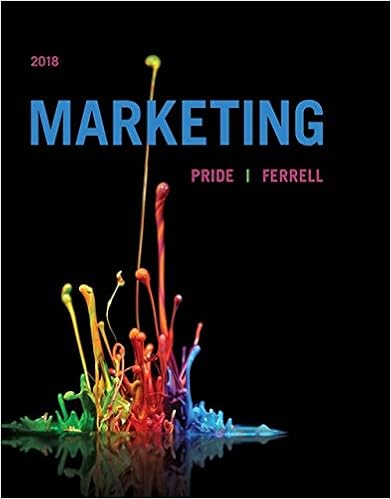# Chapter6 - 16 Which of the following is correct The...

• Notes
• 49
• 90% (10) 9 out of 10 people found this document helpful

This preview shows page 1 - 3 out of 49 pages.

##### We have textbook solutions for you!
The document you are viewing contains questions related to this textbook.The document you are viewing contains questions related to this textbook.
Chapter 19 / Exercise 6
Marketing 2018
Ferrell/PrideExpert Verified
16. Which of the following is correct? The break-even point occurs on the CVP graph where: A) total profit equals total expenses. B) total profit equals total fixed expenses. C) total contribution margin equals total fixed expenses. D) total variable expenses equal total contribution margin. Answer: C
Level: Medium LO: 1,2 17. If a company decreases its total fixed expenses while increasing the variable expense per unit, the total expense line relative to its previous position on a cost-volume-profit graph will:
Level: Medium LO: 2 18. East Company manufactures and sells a single product with a positive contribution margin. If the selling price and the variable expense per unit both increase 5% and fixed expenses do not change, what is the effect on the contribution margin per unit and the contribution margin ratio?
Level: Medium LO: 3,4 Source: CMA, adapted
##### We have textbook solutions for you!
The document you are viewing contains questions related to this textbook.The document you are viewing contains questions related to this textbook.
Chapter 19 / Exercise 6
Marketing 2018
Ferrell/PrideExpert Verified
19. Mossfeet Shoe Company is a single product firm. Mossfeet is predicting that a price increase next year will not cause unit sales to decrease. What effect would this price increase have on the following items for next year?
Level: Medium LO: 3,5 20. The contribution margin ratio is equal to: A) Total manufacturing expenses/Sales. B) (Sales - Variable expenses)/Sales. C) 1 - (Gross Margin/Sales). D) 1 - (Contribution Margin/Sales). Answer: B
Level: Medium LO: 3 21. The contribution margin ratio always increases when the:
Level: Hard LO: 3 Source: CPA, adapted 22. In the middle of the year, the price of Lake Corporation's major raw material increased by 8%. How would this increase affect the company's break-even point and margin of safety? Break-even point
Level: Easy LO: 5,7
•••